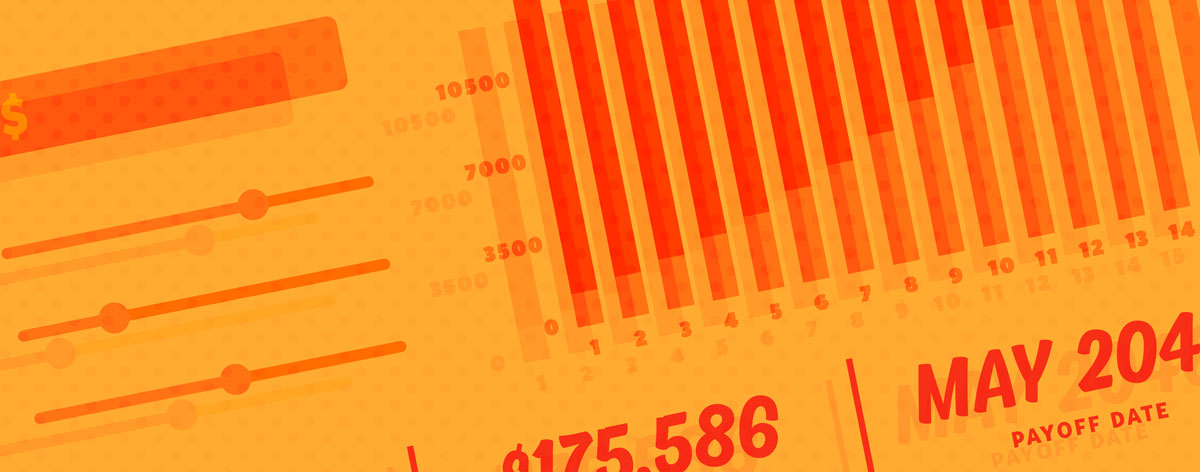Calculators
calculatorcalculatorcalculatorcalculatorcalculatorcalculatorcalculatorcalculatorcalculatorcalculatorcalculatorcalculatorcalculator